# Help needed with limiting CFrame orientation using EulerAngles and math.clamp()

I have obtained a method to make my mech’s upper body to look at the mouse as seen below for my future mech game:

``````--Obtain the offset from the hip of the mech
local rootCFrame = pointer.LowerBody.Hip.CFrame;
local rotationOffset = (rootCFrame - rootCFrame.p):inverse();

--Obtain CFrame where torso looks at the target position
local goalCFrame =  rotationOffset*CFrame.new(centerJoint,newLookAtVector)

local x,y,z = goalCFrame:ToEulerAnglesXYZ()

hipMotor.C0 = CFrame.Angles(x,y,z)
``````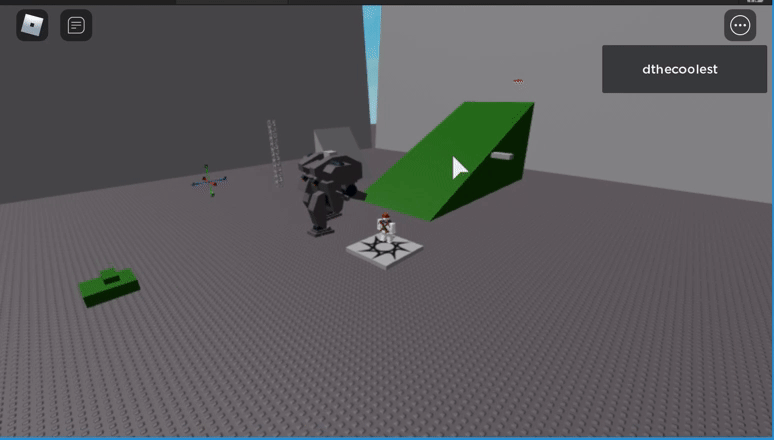Now I’m trying new methods to constrain the mechs pitch while making it be able to rotate 360° around the torso.

First I clamped the pitch which worked

``````x = math.clamp(x,-0.55,0.3)
hipMotor.C0 = CFrame.Angles(x,0,0)
``````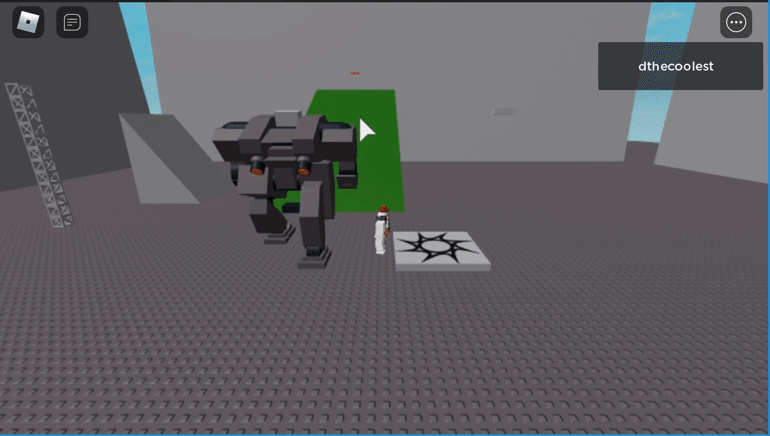The problem starts with the yaw where it just doesn’t rotate 360 degrees like I would expect.

``````hipMotor.C0 = CFrame.Angles(0,y,0)
``````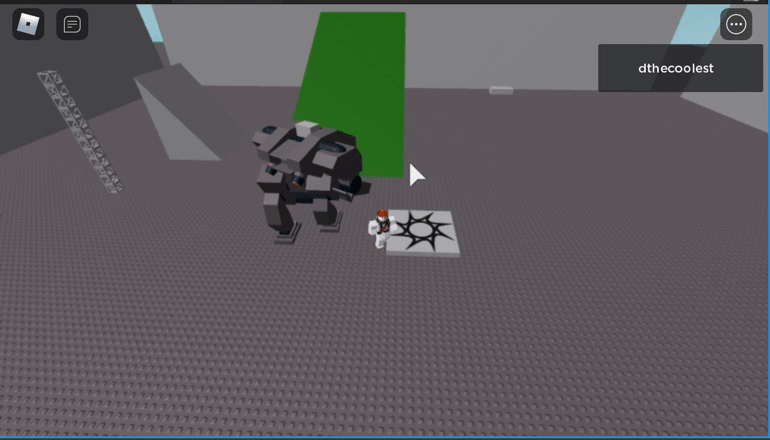Then it gets worse when combined as the torso starts rolling which is undesirable for my purposes though it looks cool.

``````hipMotor.C0 = CFrame.Angles(x,y,0)
``````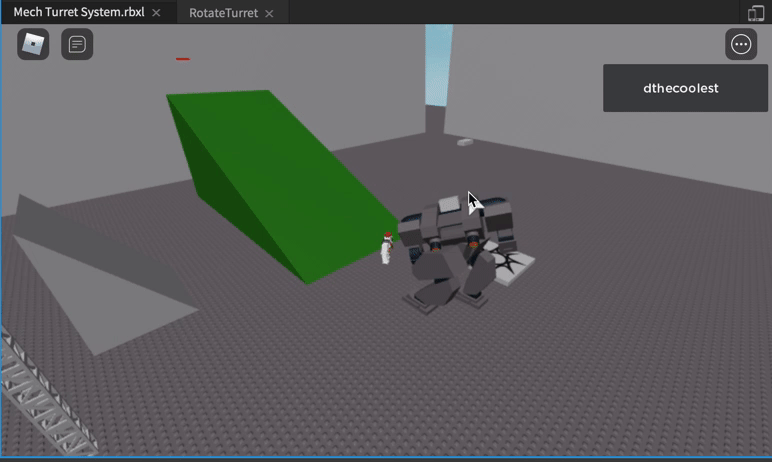I’m trying the method suggested at the end of this thread.

Please give me further insight on how to make it move like this Euler angle visualization website.
http://www.ctralie.com.s3-website-us-east-1.amazonaws.com/Teaching/COMPSCI290/Materials/EulerAnglesViz/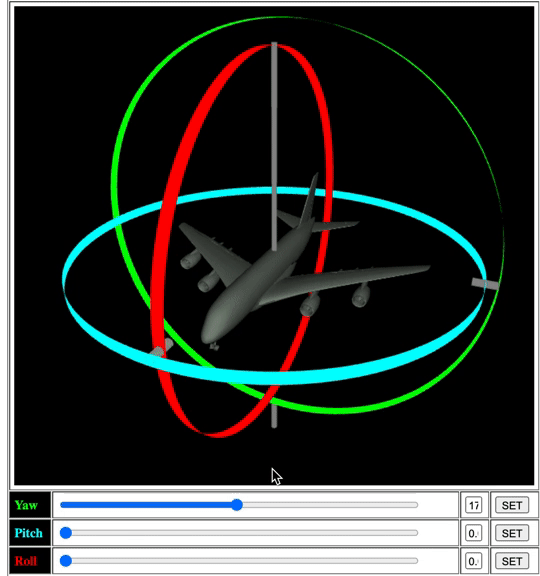1 Like

You basically want to limit it right?

If so, then you can do

Torso.CFrame:ToObjectSpace(YourCFrameCodeHere)

Hope it helps

1 Like

Sorry but I don’t think its a matter world to object relativity due to the frame already being relative the rig that I made as seen below with the selected point which is referenced to as (0,0,0) which is the centerJoint in CFrame.new() below.

``````local goalCFrame = rotationOffset *CFrame.new(centerJoint,newLookAtVector)
``````

Maybe I should just try the angle approach and get the look vector of where the legs are facing and do the if statement like that thread.

This is a really cool problem
try this:

``````local rootCFrame = pointer.LowerBody.Hip.CFrame;
local mh = mouse.Hit

hipMotor.C0 = CFrame.new(Vector3.new(), (mh.p - rootCFrame.p).Unit * Vector3.new(1, 0, 1))``````
2 Likes

It works, with a few future adjustments!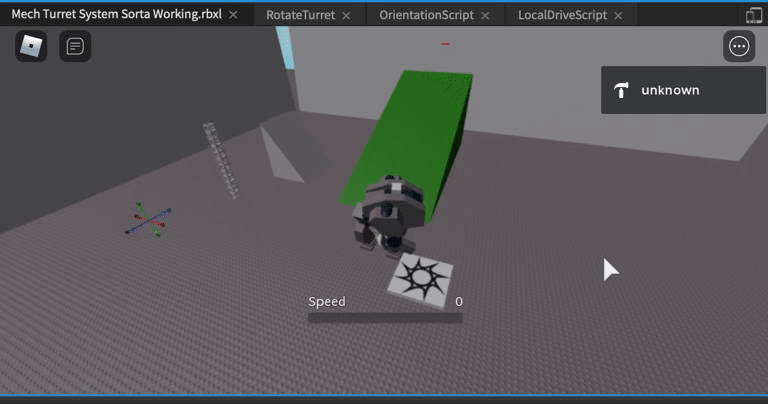Yeah this is a simple solution for disabling the y-axis of the look vector. Perhaps I should just clamp the y axis here instead of resorting to complicated Eulers angle stuff.

1 Like

For newer people viewing here is the sorta final solution of clamping the angle by finding the angle between the normal and the direction the mech is looking at. Then resolving that angle to the z-axis to find the new look direction vector. Unfortunately, it breaks when you look directly down at your feet so maybe more clamping will solve the issue.

``````--Obtained where torso looks at the target position
local x = newLookAtVector.Unit.X
local y = newLookAtVector.Unit.Y
local z = newLookAtVector.Unit.Z

--Obtain the normal of where the turret is mounted on
local baseNormal = pointer.LowerBody.Hip.CFrame.UpVector

--angle of look direction and the normal of the floor is
local angleToNormal = math.acos( baseNormal:Dot(newLookAtVector.Unit)/(baseNormal.Magnitude * newLookAtVector.Unit.Magnitude) )
--obtain angle to horizontal to find the angle of elevation and depression
local horizontalAngle = math.pi/2-angleToNormal
--clamp the angle of depression or elevation
horizontalAngle = math.clamp(horizontalAngle,-depressionAngle ,elevationAngle)
--convert back to an angle between normal vector and the look vector
angleToNormal = math.pi/2-horizontalAngle
print(angleToNormal)
--Resolve the angle to
local newY = 1*math.cos(angleToNormal)
local newLook = Vector3.new(x,newY,z)
local goalCFrame = rotationOffset*CFrame.new(Vector3.new(),newLook)

hipMotor.C0 = goalCFrame*CFrame.new(0,yOffset,0)
``````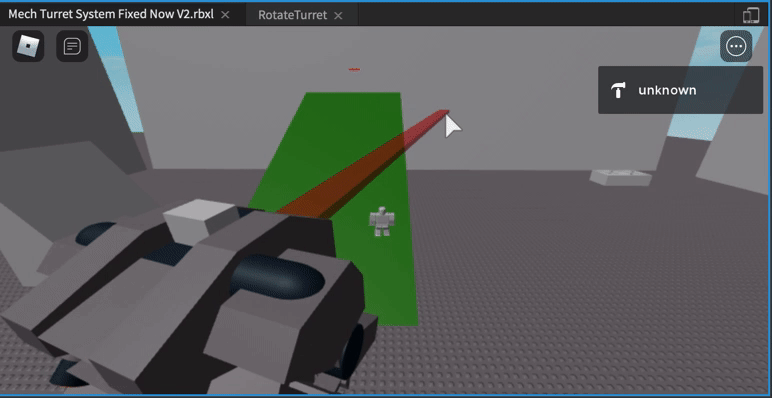3 Likes